# Math Tuition## Unlocking Your Child’s Potential with Odyssey Math – Singapore’s Premier Math Tuition

Unleashing the Power of Math in Today’s Dynamic World Mathematics is required for numerous industries, from science and technology to engineering and finance. A strong grasp of math concepts is paramount for students to excel academically and flourish in their careers. Meet Odyssey Math : Singapore’s Premier Math Tuition Odyssey Math, founded by Mr Justin …## Inspiring Your Kids to Love and Excel in Math

Igniting a belief to do well for Mathematics: The Ultimate Guide for Singapore Parents Many parents send their kids to my math tuition center, telling me that their kids did not do well for mathematics. Reasons that they gave were laziness, unwillingness to do homework, falling behind classes and not understanding the math concepts taught …Elementary Math, is it difficult? Elementary Mathematics built on math concepts learnt in the previous years in secondary school. E Math is not difficult if students consistently revise the concepts they have learnt and practice more questions. When it comes to E Math, the most important thing that students need is intuition. This can be …Additional Math, is it difficult? Additional Mathematics is quite different from Elementary Mathematics. You still require the usual algebraic manipulation skills you have learnt in E Math to do A Math, but A Math has more formula to use. The way I see it, A Math is not difficult if you know when and how …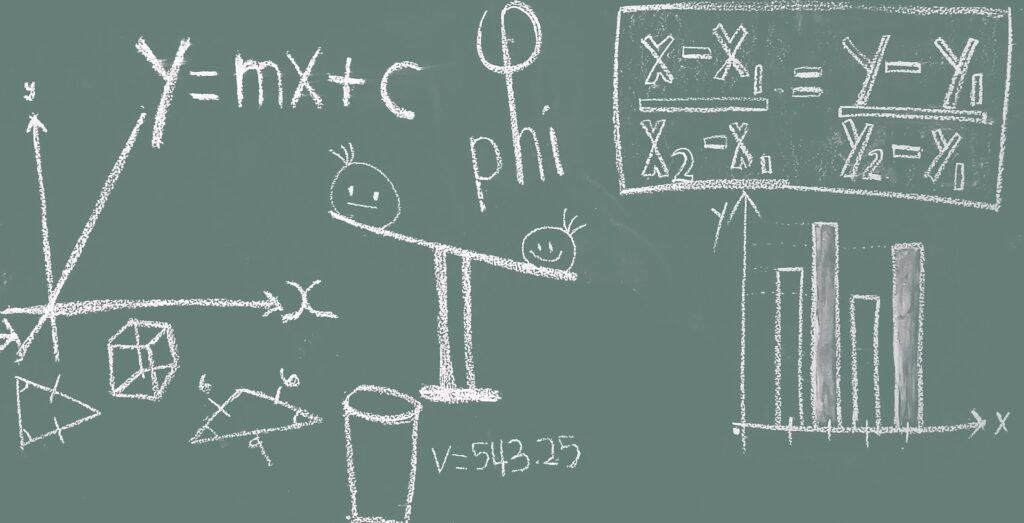How Important Is Secondary 2 Math? As a parent, witnessing your child navigate Secondary 2 math in Singapore can be a nail-biting experience. This is particularly true when the results for Secondary 2 math will determine whether your child is able to take Additional Mathematics.  This is pretty much an important subject especially for students …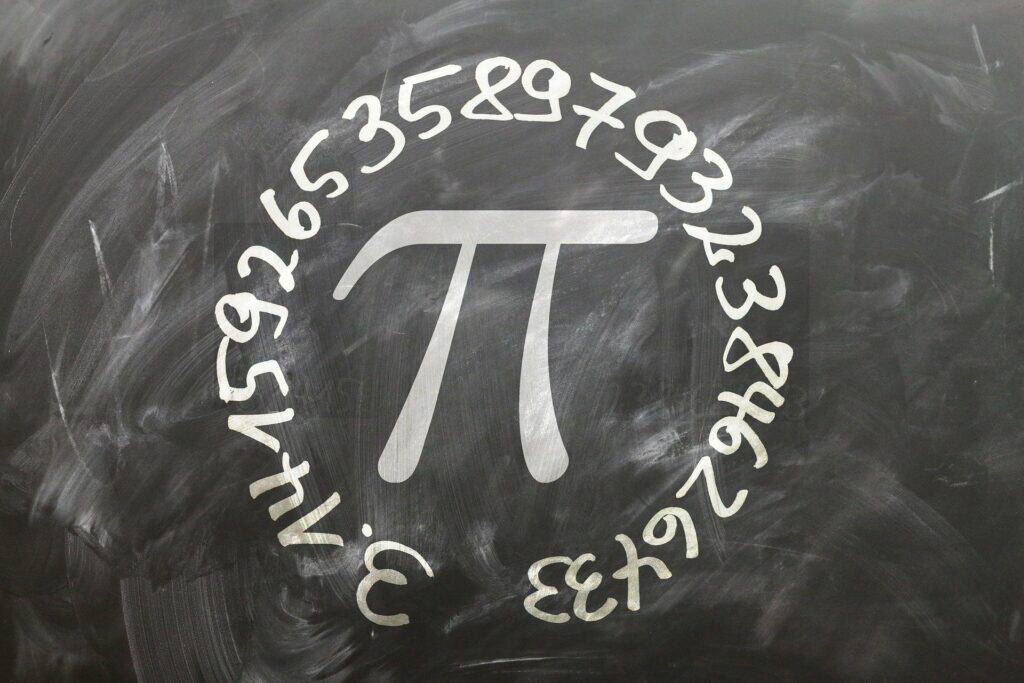Secondary 1 marks a pivotal milestone for Singaporean students, where they moved on from primary school math and embark their journey on secondary school math. It is important to check in with your child from time to time on how he/she is coping with secondary math. One thing is for certain, they will have to …For many students embarking their journey in Junior College, the experience of taking Junior College A Level H2 Mathematics is vastly different as compared to O Level Additional and Elementary Mathematics. The content for Junior College Math is so much more, coupled with the fact that questions are going to be more difficult and learning …## 9 Famous Mathematicians In History

Meet some of the famous mathematicians who have transformed the realm of mathematics and made contribution to the world with their ingenuity. Mathematical discovery is more about curiosity of the human mind rather than talent. The contributions made by these famous mathematicians have positive impacts on disciplines like physics, engineering and computer science. Euclid of …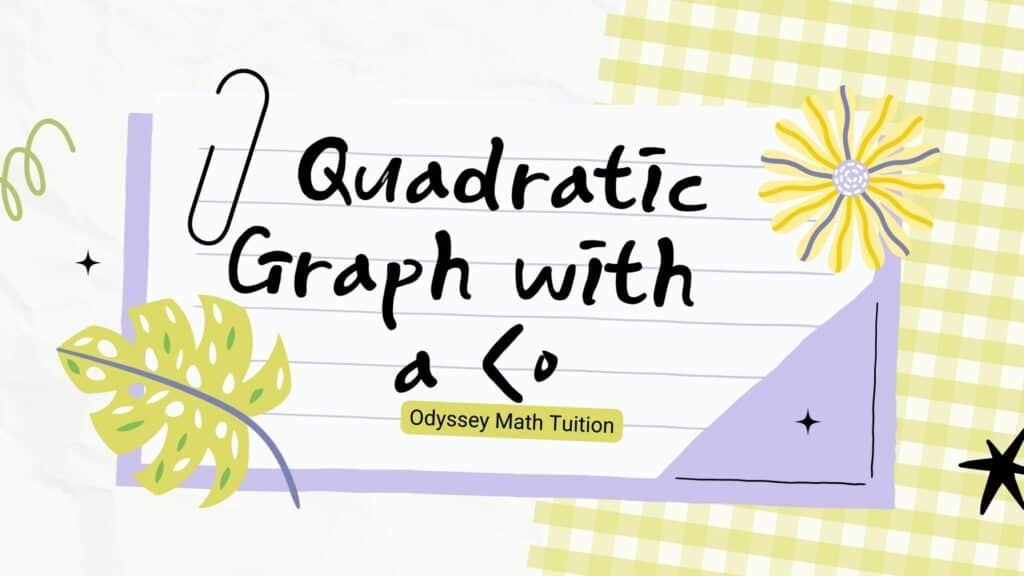## Conditions for a quadratic graph to be always negative when a<0

We know that when the coefficient a is less than 0, the quadratic graph is a frowning curve. In order for this frowning quadratic graph to be always negative, meaning it will always be below the x-axis, what this also means is that the curve does not cut the x-axis. Therefore, to find the condition, …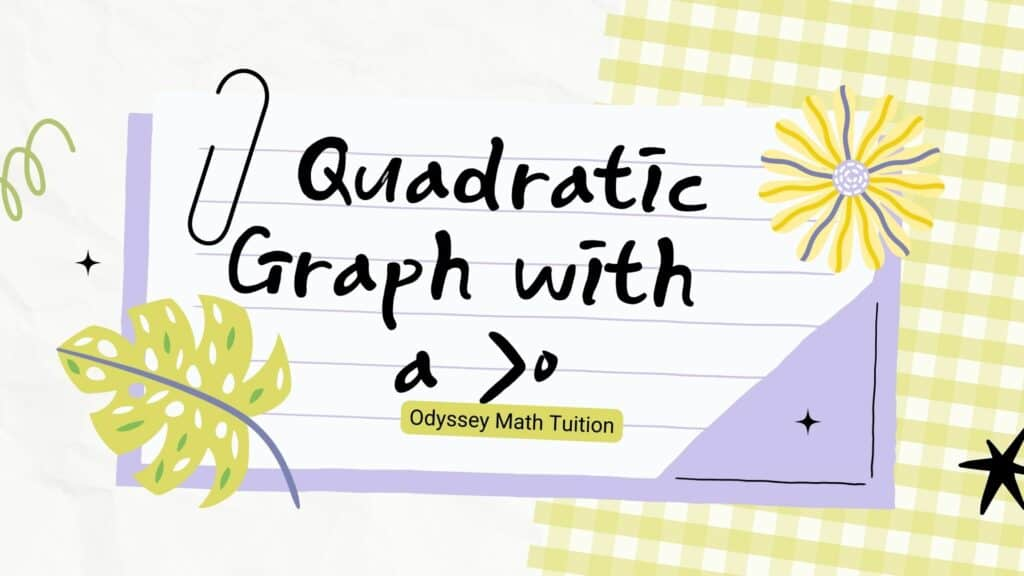## Conditions for a quadratic graph to be always positive when a>0

We know that when the coefficient a is more than 0, the quadratic graph is a smiling curve. In order for this smiling quadratic graph to be always positive, meaning it will always be above the x-axis, what this also means is that the curve does not cut the x-axis. Therefore, to find the condition, …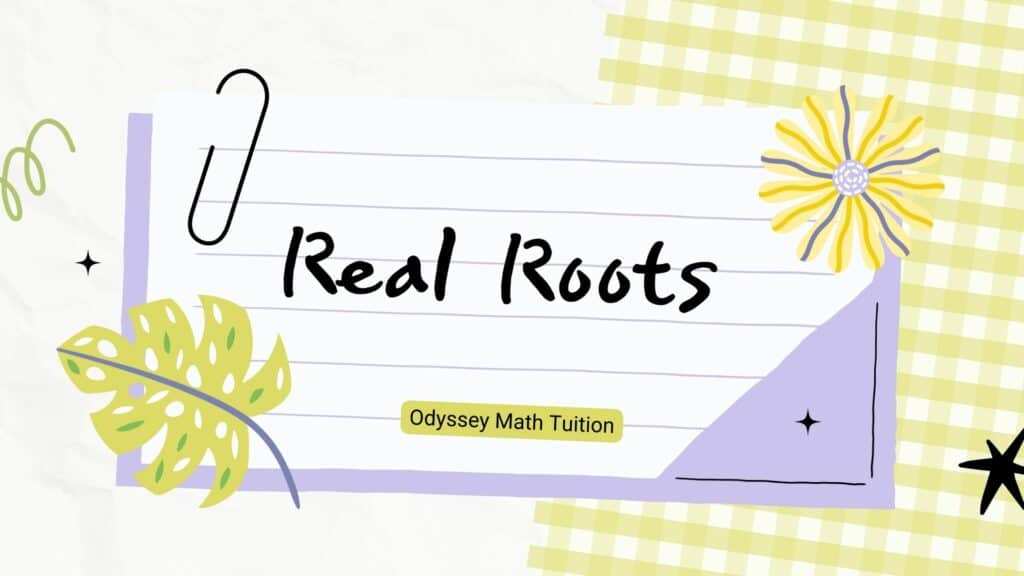## Conditions for a quadratic equation to have real roots

For a quadratic equation to have real roots, discriminant must be more than or equal 0. Note the emphasize on “more than or equal to”. Set up this equality and solve it, you will get the answer. One important thing to note is that for real roots, it also means that a line intersects a given curve. …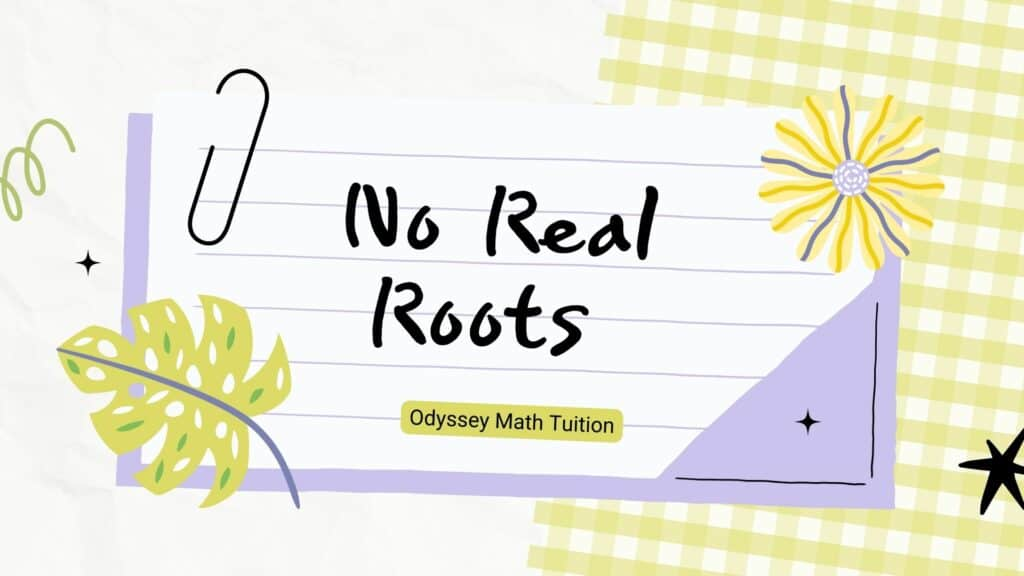## Conditions for a quadratic equation to have no real roots

For a quadratic equation to have no real roots, discriminant must be less than 0. Set up this equality and solve it, you will get the answer. One important thing to note is that for no real roots, it also means that a line does not intersect a given curve. Let’s move on to understand more about real …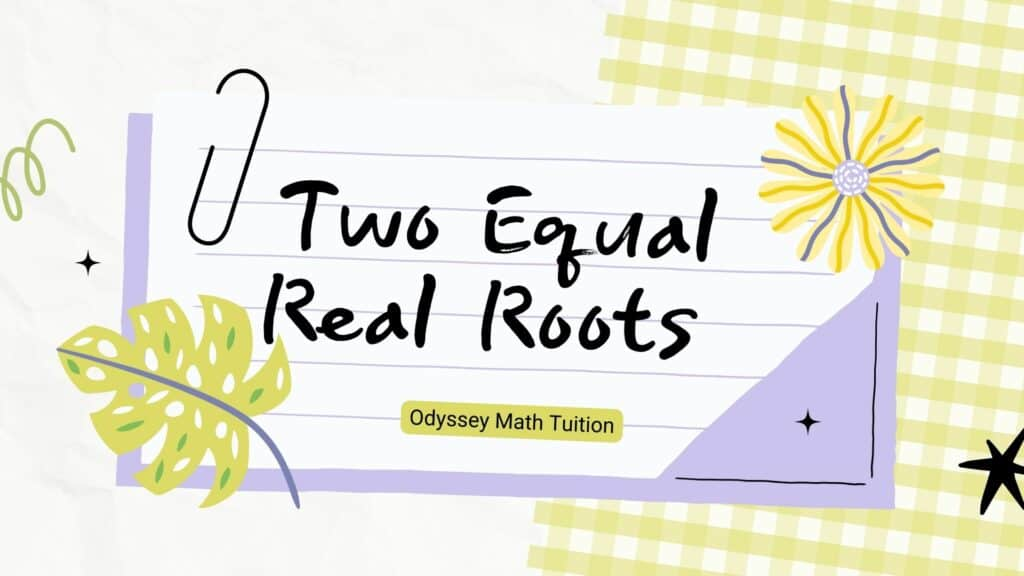## Conditions for a quadratic equation to have two equal real roots

For a quadratic equation to have two equal real roots, discriminant must be equal to 0. Set up this equality and solve it, you will get the answer. One important thing to note is that for two equal real roots, it also means that a line does not  Let’s move on to understand more about no real roots. …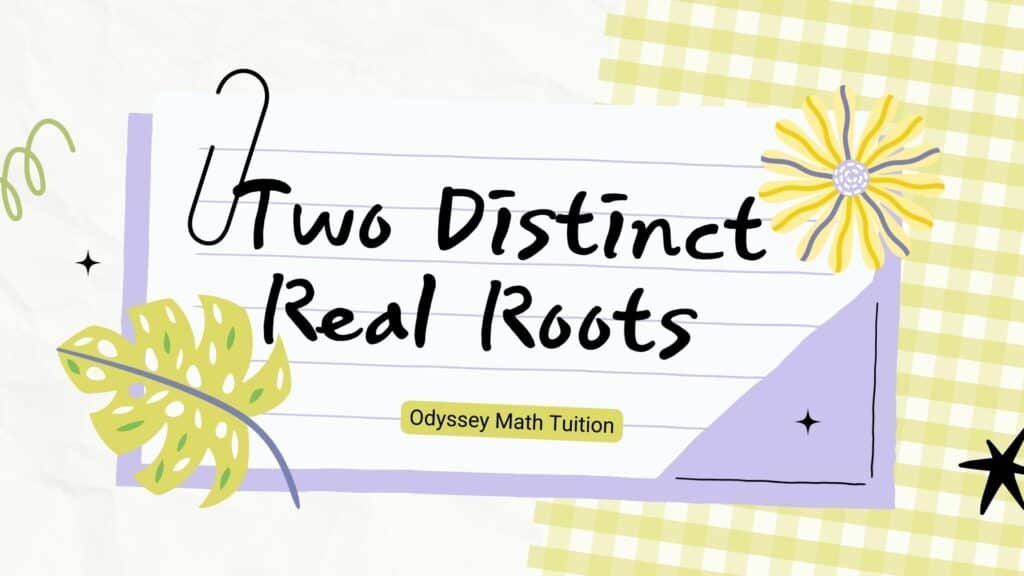## Conditions for a quadratic equation to have two distinct real roots

For a quadratic equation to have two distinct real roots, discriminant must be more than 0. Set up this inequality and solve it, you will get the answer. One important thing to note is that for two distinct real roots, it also means that a line intersects a given curve at two points. Let’s move …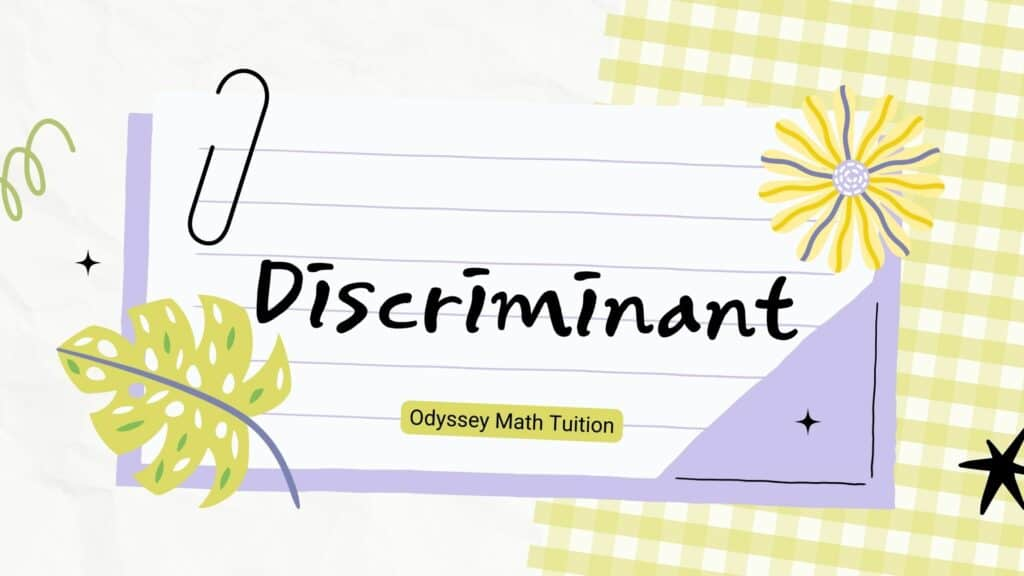## Discriminant of a Quadratic Equation

Discriminant of a Quadratic Equation is a new concept that you have to learn in Secondary 3 Additional Mathematics. This concept will be tested over and over again in exams and in this post, you will understand the fundamentals of discriminant. A very quick summary on what you will be learning here: 1) If discriminant …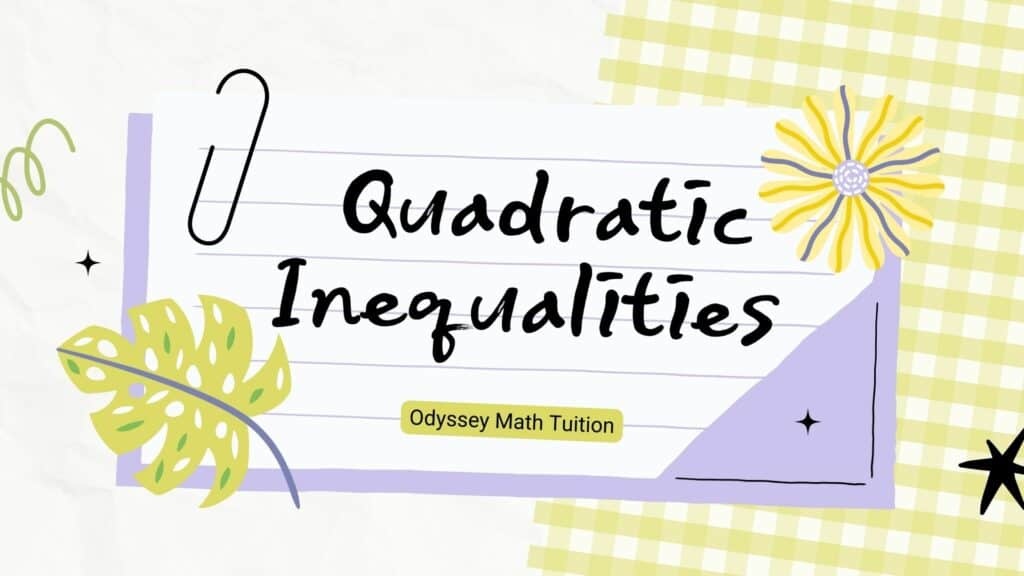## Solving Quadratic Inequalities Part 2

Previously, you have learnt how to solve quadratic inequalities where the portion of the curve is below the x-axis. In this post, you will be learning how to solve quadratic inequalities where the portion of the curve is above the x-axis. Not to worry, the solving style is the same, so there is not much new …## Solving Quadratic Inequalities Part 1

Earlier, you have recapped on basic inequalities. In this post, you will learn how to solve quadratic inequalities where the portion of the curve is below the x-axis. Now that you have learnt how to solve quadratic inequalities for the portion of the curve below the x-axis, let’s move on to solve quadratic inequalities where the …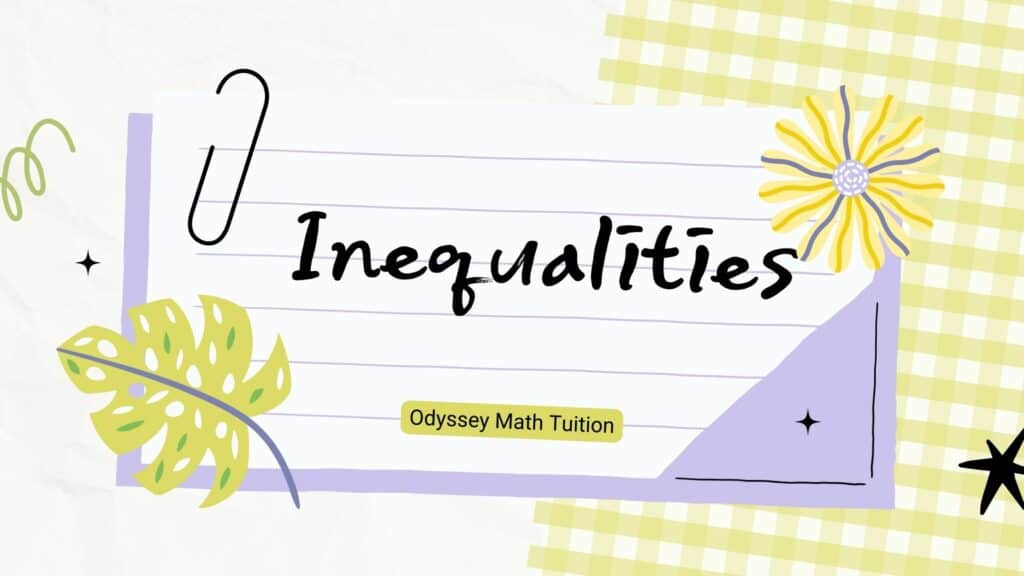## Inequalities

Inequalities is a must to recap, since you would dabble with a lot of inequalities in this chapter. Let’s do a quick revision of basic inequalities before we proceed further in this chapter. With the understanding of basic inequalities, let’s now learn how to solve quadratic inequalities. If you are looking for math tuition, please visit Odyssey Math …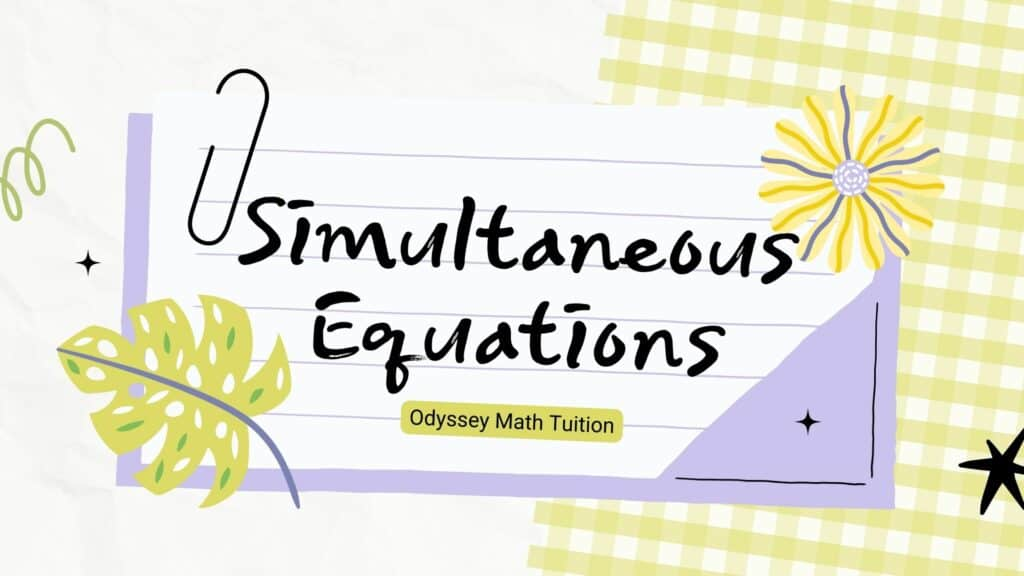## Simultaneous Equations

Simultaneous Equations are two or more algebraic equations that share variables, quite commonly, x and y. In this post, you will understand how to solve simultaneous equations involving non linear equations using the substitution method. Congratulations on understanding Simultaneous Equations! Follow this post next to learn more about Quadratic Equations and its characteristics. If you are …## How to know if the math tuition your child is attending is good

Understandably, parents will always be wondering if the math tuition their child is attending is good. They will be thinking if their child’s results will improve after attending tuition. How to know if the math tuition is good? Here are 4 ways that you can gauge whether the math tuition is good for your child. 1) …## 5 things students must do to maximize their learning in math tuition

Going to Math Tuition does not necessarily mean that students will be able to rectify their misconceptions in mathematics as well as improve their understanding. As much as we tutors can do our best to provide the best guidance, resources and explanation, students are required to do their part in terms of learning as well. …## 4 Key Benefits of Math Tuition

What are the 4 key benefits of Math Tuition? Dear parents, are you questioning whether sending your kids to additional lessons (math tuition) will help improve their results?  After all, lessons at schools sometimes does not seem to help your kids in scoring well in their exams. Let me provide you in details how Math Tuition …## Is Math Tuition Important?

Are students always lamenting that they could not keep up with math concepts taught in class? Are students struggling to keep pace which resulted in their lack of understanding for math concepts which resulted in them failing math exams? These are some of the common reasons why students are unable to do well in mathematics. …